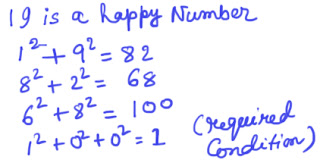How to find Happy Number in JAVA?

0
19
• 1
•
•
•
•
•
•
•
•
•
1
ShareWhat is Happy Number:

Definition of Happy Number:

To find out whether a number is happy, square each of its digits, add the answers and repeat. If you end up with 1 the number is happy!

Logic for Happy Number:

We start with some positive integer.We then replace the number by the sum of the squares of its digits, and repeat the process until the number equals 1 , or it loops in a cycle until it gets some number that equals a number obtained in previous calculation which does not include 1. Those numbers for which this process ends in 1 are happy numbers, while those that do not end in 1 are unhappy numbers .

Example: 19 is a happy number asHappy number

Likewise 1, 7, 10, 13, 19, 23 are happy numbers.

Algorithm:

1. First we need to take two numbers as input: they are actually the ranges between which the Happy Numbers are to be found out.
The first ‘for loop’ is used to tackle this range.
2.If the sum of the digits of the number is equal to 1, it is a happy number.
3. But if sum of the digits of the number is not equal to 1, then two conditions can happen:
a> We can get some number which is already obtained in some previous calculation.This is the terminating condition.
b>We can go on finding the sum of the digits of the number as long as condition 2 or 3 is not satisfied.

while(s!=1) takes care of this.

4.Then we summed up the digits of the number and check whether it is already obtained from the string. If not obtained before it is stored in a string where + is used as a delimiter.

Code for Happy Number

``````
import java.io.*;
public class Happy2
{
public static void main(String args[])
{
try
{
Second sec=new Second();
sec.fun();
}
catch(Exception e)
{
System.out.println(e);
}
}
}
class Second
{
String st,en,ct;
int st1,en1,r,s,f,no,i1,flag;
char ch[];
public void fun() throws Exception
{
System.out.println("Enter the Starting range");
//Take starting number from user
st1=Integer.parseInt(st); //Convert the string into integer
System.out.println("Enter the Ending range"); //Take ending range from user
en1=Integer.parseInt(en);  //Convert the string into integer
for(int i=st1;i<=en1;i++)
{
flag=0;
s=0;
ct="";
i1=i;
while(s!=1)
//The string terminates if 1 is obtained
{
flag=0;
s=0;
while(i1>0)
//to get the square of every digit of the number
{
r=i1%10;//finding the remainder
f=1;
f=r*r;
s=s+f;
//storing the sum of square of every digit
i1=i1/10;
//finding the quotient of every number
}
i1=s;
ch=ct.toCharArray();//convert the string into array
no=0;
for(int k=0;k<ch.length;k++)
//checking whether the number obtained after summing up every digit of the number is already present
{
if(ch[k]>=48 && ch[k]<=57) //checking whether it is a digit
{
no=no*10+(int)ch[k]-48;//converting the character into digit
}
else
{

if(no==s)//if digit obtained is same as the digit obtained in some previous case
{
flag=1;//then we set a flag value as 1
no=0;
break;
}
no=0;
}
}
if(flag==1)
break;
ct=ct+s+"+";
}
if(flag==0)//if s=1 and if digit obtained is not same as the digit obtained in some previous case
System.out.println(i);
for(int k=0;k<ch.length;k++)
{
ch[k]='';//sets the string to null
}
}
}
}
``````
Don't miss out!

Receive top technical news, lesson ideas, travel tips and more!

Give it a try. You can unsubscribe at any time.